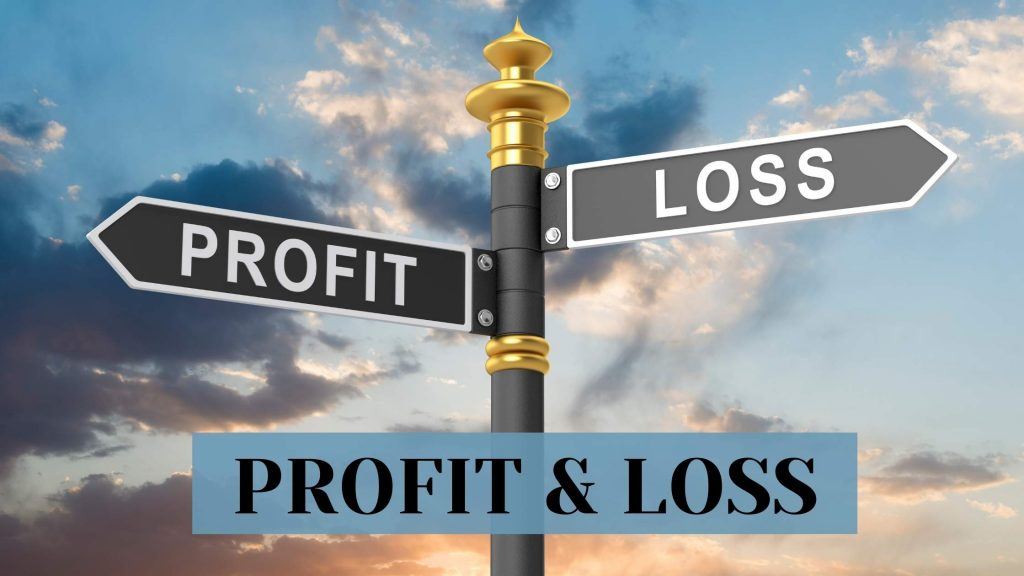Maths
Study Material

# Profit and Loss

In today’s world, each and every company (or business) works on the basic fundamental concept of profit and loss. The term 'Profit and Loss' is a real-life concept developed from various applications which take place in our day-to-day lives.

4 minutes long
Posted by Kunduz Tutor, 26/11/2021Hesap Oluştur

Got stuck on homework? Get your step-by-step solutions from real tutors in minutes! 24/7. Unlimited.

In today’s world, each and every company (or business) works on the basic fundamental concept of profit and loss. The term ‘Profit and Loss’ is a real-life concept developed from various applications which take place in our day-to-day lives. Here, we will read about the profit loss formula.

Author – Ojasvi Chaplot

## Profit and Loss Formula

• The basic formula for calculating the profit is:

PROFIT (P) = SELLING PRICE (S.P.) – COST PRICE (C.P.)

•  The basic formula for calculating the loss is:

LOSS (L) = COST PRICE (C.P.) – SELLING PRICE (S.P.)

## General Terminologies of Profit and Loss Formula

We come across the term profit and loss a number of times. When someone buys a product for a particular price and sells it at a different price, then either a profit is made or a loss is incurred.

There are various terms that are associated with profit and loss formula; like: cost price (C.P.), selling price (S.P.), discount, marked price, profit, and loss.

So, to become more familiar with these terms and concept of profit and loss formula, let us see some terminologies related to the profit and loss formula.

### Cost Price (C.P.)

The cost price of a product is the price at which the product is purchased. It can also be defined as the amount paid to purchase the commodity. Cost price is denoted as C.P.

For Example – If Ojasvi bought an umbrella for \$10; so the cost price of the umbrella is \$10 (i.e. C.P. of Umbrella = \$10).

### Selling Price (S.P.)

The selling price of a product is the price at which the product is sold. It can also be defined as the amount to sell the commodity. Selling price is denoted as S.P.

For Example – If Ojasvi sold the umbrella for \$12; so the selling price of the umbrella is \$12 (i.e. S.P. of Umbrella = \$12).

### Profit (P) or Gain

The amount that is gained by selling a product is termed as profit or gain. The profit is gained when the selling price of a product is greater than its cost price.

The Profit or Gain is calculated as:

PROFIT (P) = SELLING PRICE (S.P.) – COST PRICE (C.P.)

For Example – If Ojasvi bought an umbrella for \$10 and sold it for \$12, then the profit gained by Ojasvi on the umbrella is \$2 (i.e. Profit = S.P. of Umbrella – C.P. of Umbrella = \$12 – \$10). Also, the profit percentage, %P will be 20% (i.e. %P = [Profit/C.P.] x 100 = (2/10) x 100 = 200/10).

### Loss(L)

The amount that is lost by selling a product is termed as loss. The loss is incurred when the cost price of a product is greater than its selling price.

The formula of Loss is given as:

LOSS (L) = COST PRICE (C.P.) – SELLING PRICE (S.P.)

For Example – Now, let us consider that if Ojasvi bought the umbrella for \$10 and sold it for \$8, then the loss incurred to Ojasvi on the umbrella is \$2 (i.e. Loss = C.P. of Umbrella – S.P. of Umbrella = \$10 – \$8). Also, the loss percentage, %L will be 20% (i.e. %L = [Loss/C.P.] x 100 = (2/10) x 100 = 200/10).

### Discount

In a business, so as to cope up with the competition and boost the sale of goods, the shopkeepers offer discounts to the customers. The offers or the rebate given by the shopkeepers which lure and attract the customers to purchase the products is called a discount. It is always calculated on the marked price (M.P.) of the product.

Marked Price (M.P.) is the price usually labelled by the shopkeeper, for offering the discount to the customers.

Discount Formula in Profit and Loss:

• DISCOUNT = Marked Price (M.P.) – Selling Price (S.P.)
• Discount (%) or Percentage Discount = ( Discount / Marked Price ) × 100

For Example – If the marked price (M.P.) of a product is \$100, and there is a 20% discount on it, so the customer can buy the product at the following price:

20% discount on marked price (M.P.) of the product = (20/100) × 100

Discount = 2000/100 = \$20

Therefore, Selling Price (S.P.) = Marked Price (M.P.) – Discount = \$100 − \$20 = \$80

## Profit and Loss Percentage Formula

After the profit and loss is calculated, sometimes we need to calculate the profit and loss in terms of percentage. This helps in comparing two quantities.

• Profit Percentage Formula

PROFIT PERCENTAGE (%P) = (PROFIT/C.P.) x 100 = [(S.P. – C.P.)/C.P.] x 100

• Loss Percentage Formula

LOSS PERCENTAGE (%L) = (LOSS/C.P.) x 100 = [(C.P. – S.P.)/C.P.] x 100

## Profit and Loss Formula and Tricks

S.P. = {(100 + %P)/100} × C.P. ; if SP > CP

S.P. = {(100 – %L)/100} × C.P. ; if SP < CP

C.P. = {100/(100 + %P)} × S.P. ; if SP > CP

C.P. = {100/(100 – %L)} × S.P. ; if SP < CP

## Picked For You

Furthermore, these are some topics which might interest you: